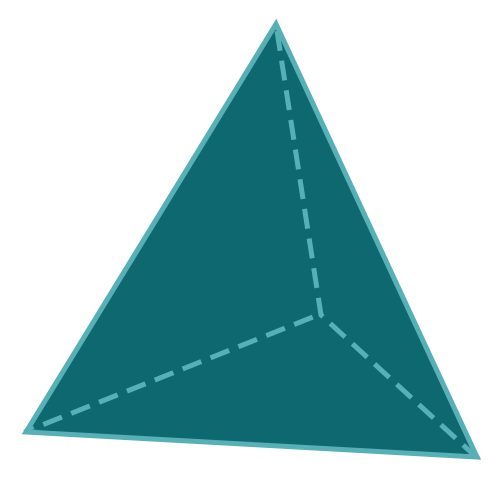Report a Problem
Suggestions

#Calculate The Surface Area of A Tetrahedron Pyramid

Last updated: Saturday, April 29, 2023
Select a type of pyramid below
Rectangular Base
Square Base
Equilateral Triangular Base
Tetrahedron
Pentagonal Base
Hexagonal Base

A tetrahedron pyramid is a four-sided pyramid with a triangular base. It is a fundamental shape in geometry and is widely used in various fields of science, engineering, and mathematics. The surface area of a tetrahedron pyramid can be calculated using the formula shown below.

The tetrahedron pyramid has unique structural properties, making it useful in various applications. In construction, it can be used as a base for buildings, bridges, and other structures, as its shape provides stability and strength. In mathematics, the tetrahedron pyramid is used to solve complex geometric problems, such as calculating the volume of irregular shapes.

The tetrahedron pyramid also has applications in physics, particularly in crystallography, where it is used to describe the structure of certain types of crystals. In chemistry, the tetrahedron pyramid is used to describe the structure of certain types of molecules, such as methane, which has a tetrahedral structure.

Overall, the tetrahedron pyramid is a fascinating shape that has a wide range of applications in science, engineering, and mathematics. Its unique properties make it an essential tool for solving complex problems and understanding the world around us.

The formula for determining the surface area of a tetrahedron pyramid is defined as:
$$SA$$ $$=$$ $$\sqrt{3}$$ $$\cdot$$ $$a^2$$
$$SA$$: the surface area of the pyramid
$$a$$: the length of any side
The SI unit of surface area is: $$square \text{ } meter\text{ }(m^2)$$

## Find $$SA$$

Use this calculator to determine the surface area of a tetrahedron when the length of any side is given.
Hold & Drag
CLOSE
the length of any side
$$a$$
$$meter$$
Bookmark this page or risk going on a digital treasure hunt again